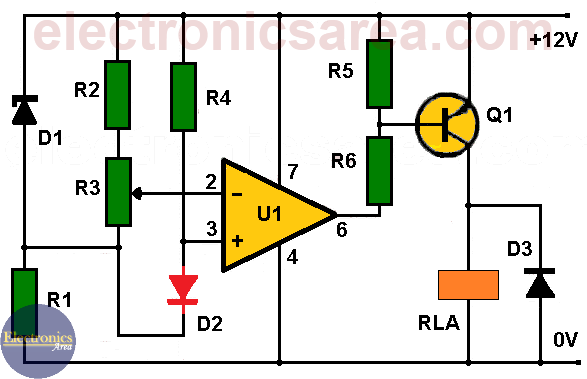# Over-temperature alarm using operational amplifier

On the diagram below, you can see how a semiconductor diode is used as a temperature sensing element in an over-temperature alarm using operational amplifier.

Semiconductor diodes can be used as temperature sensors, because they change their forward voltage with the change in temperature.

The advantage that diodes have is that their relation between temperature and voltage is practically linear.

## How the over-temperature alarm using operational amplifier works?

On this circuit the Zener diode is connected in series with the resistor R1, so that between the ends of the two voltage dividers formed by R2-R3 and R4-D1, there is a constant voltage of 5.6 V. Practically a constant current circulates for each of these dividers.So, we have:

• A constant reference voltage between the R2 and R3 resistors junction and the # 2 pin of the operational amplifier, and
• A temperature-dependent voltage with a coefficient of -2 mV /°C between the R1 – R3 resistors junction and the # 3 pin of the operational amplifier.

Therefore, a differential voltage with a coefficient of -2 mV/°C appears between pins 2 and 3 of the amplifier. To tune this circuit, the D2 diode is raised to the desired temperature level, and potentiometer R3 is slowly set , just before the relay is activated.

At this time between pins 2 and 3 of the operational amplifier appears a differential voltage of 1 mV, with the voltage at pin 3 below the voltage at pin 2, which activates transistor Q1 and relay.

When the temperature drops below the trigger level, the pin 3 voltage increases above the pin 1 voltage at about 2 mV/°C. Whereby the transistor Q1 and the relay are de-energized.

The circuit has a typical sensitivity of 0.5 °C, and can be used as an overtemperature alarm that works from below zero temperatures (0°C) to above the boiling point temperature of the water (100°C).

The operation of the circuit can be reversed to act as an insufficient temperature alarm by reversing the pin 2 and pin 3 connections of the operational amplifier.

## Over-temperature alarm list of components

• 1  741 operational amplifier (U1)
• 1  2N3702 PNP transistor (Q1)
• 1  5.6 V Zener diode (D1)
• 2  common semiconductor diodes (D2, D3)
• 1  9V relay (RLA)
• 3  1.2k resistors (R1, R5, R6)
• 2  4.7k resistors (R2, R4)
• 1  1k potentiometer (R3)
• 2
•
• 27
•
•
•
•
•
29
Shares
•
29
Shares
• 2
•
• 27
•
•
•
•